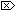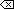The Open Group Base Specifications Issue 6
IEEE Std 1003.1-2001

#### NAME

fmod, fmodf, fmodl - floating-point remainder value function

#### SYNOPSIS

```#include <math.h> double fmod(double x, double y); float fmodf(float x, float y); long double fmodl(long double x, long double y); ```

#### DESCRIPTION

[CX]The functionality described on this reference page is aligned with the ISO C standard. Any conflict between the requirements described here and the ISO C standard is unintentional. This volume of IEEE Std 1003.1-2001 defers to the ISO C standard.These functions shall return the floating-point remainder of the division of x by y.

An application wishing to check for error situations should set errno to zero and call feclearexcept(FE_ALL_EXCEPT) before calling these functions. On return, if errno is non-zero or fetestexcept(FE_INVALID | FE_DIVBYZERO | FE_OVERFLOW | FE_UNDERFLOW) is non-zero, an error has occurred.

#### RETURN VALUE

These functions shall return the value x- i* y, for some integer i such that, if y is non-zero, the result has the same sign as x and magnitude less than the magnitude of y.

If the correct value would cause underflow, and is not representable, a range error may occur, and [MX]either 0.0 (if supported), oran implementation-defined value shall be returned.

[MX]If x or y is NaN, a NaN shall be returned.

If y is zero, a domain error shall occur, and either a NaN (if supported), or an implementation-defined value shall be returned.

If x is infinite, a domain error shall occur, and either a NaN (if supported), or an implementation-defined value shall be returned.

If x is ±0 and y is not zero, ±0 shall be returned.

If x is not infinite and y is ±Inf, x shall be returned.

If the correct value would cause underflow, and is representable, a range error may occur and the correct value shall be returned.#### ERRORS

These functions shall fail if:

Domain Error
[MX]The x argument is infinite or y is zero.

If the integer expression (math_errhandling & MATH_ERRNO) is non-zero, then errno shall be set to [EDOM]. If the integer expression (math_errhandling & MATH_ERREXCEPT) is non-zero, then the invalid floating-point exception shall be raised.These functions may fail if:

Range Error
The result underflows.

If the integer expression (math_errhandling & MATH_ERRNO) is non-zero, then errno shall be set to [ERANGE]. If the integer expression (math_errhandling & MATH_ERREXCEPT) is non-zero, then the underflow floating-point exception shall be raised.

The following sections are informative.

None.

#### APPLICATION USAGE

On error, the expressions (math_errhandling & MATH_ERRNO) and (math_errhandling & MATH_ERREXCEPT) are independent of each other, but at least one of them must be non-zero.

None.

#### FUTURE DIRECTIONS

None.

feclearexcept() , fetestexcept() , isnan() , the Base Definitions volume of IEEE Std 1003.1-2001, Section 4.18, Treatment of Error Conditions for Mathematical Functions, <math.h>

#### CHANGE HISTORY

First released in Issue 1. Derived from Issue 1 of the SVID.

#### Issue 5

The DESCRIPTION is updated to indicate how an application should check for an error. This text was previously published in the APPLICATION USAGE section.

#### Issue 6

The behavior for when the y argument is zero is now defined.

The fmodf() and fmodl() functions are added for alignment with the ISO/IEC 9899:1999 standard.

The DESCRIPTION, RETURN VALUE, ERRORS, and APPLICATION USAGE sections are revised to align with the ISO/IEC 9899:1999 standard.

IEC 60559:1989 standard floating-point extensions over the ISO/IEC 9899:1999 standard are marked.

End of informative text.

UNIX ® is a registered Trademark of The Open Group.
POSIX ® is a registered Trademark of The IEEE.
[ Main Index | XBD | XCU | XSH | XRAT ]